# Fraction calculator

The calculator performs basic and advanced operations with fractions, expressions with fractions combined with integers, decimals, and mixed numbers. It also shows detailed step-by-step information about the fraction calculation procedure. Solve problems with two, three, or more fractions and numbers in one expression.

## Result:

### 32/5 + 34/6 = 106/15 = 7 1/15 ≅ 7.0666667

Spelled result in words is one hundred six fifteenths (or seven and one fifteenth).

### How do you solve fractions step by step?

1. Conversion a mixed number 3 2/5 to a improper fraction: 3 2/5 = 3 2/5 = 3 · 5 + 2/5 = 15 + 2/5 = 17/5

To find new numerator:
a) Multiply the whole number 3 by the denominator 5. Whole number 3 equally 3 * 5/5 = 15/5
b) Add the answer from previous step 15 to the numerator 2. New numerator is 15 + 2 = 17
c) Write a previous answer (new numerator 17) over the denominator 5.

Three and two fifths is seventeen fifths
2. Conversion a mixed number 3 4/6 to a improper fraction: 3 4/6 = 3 4/6 = 3 · 6 + 4/6 = 18 + 4/6 = 22/6

To find new numerator:
a) Multiply the whole number 3 by the denominator 6. Whole number 3 equally 3 * 6/6 = 18/6
b) Add the answer from previous step 18 to the numerator 4. New numerator is 18 + 4 = 22
c) Write a previous answer (new numerator 22) over the denominator 6.

Three and four sixths is twenty-two sixths
3. Add: 17/5 + 22/6 = 17 · 6/5 · 6 + 22 · 5/6 · 5 = 102/30 + 110/30 = 102 + 110/30 = 212/30 = 2 · 106/2 · 15 = 106/15
For adding, subtracting, and comparing fractions, it is suitable to adjust both fractions to a common (equal, identical) denominator. The common denominator you can calculate as the least common multiple of both denominators - LCM(5, 6) = 30. In practice, it is enough to find the common denominator (not necessarily the lowest) by multiplying the denominators: 5 × 6 = 30. In the next intermediate step, , cancel by a common factor of 2 gives 106/15.
In words - seventeen fifths plus twenty-two sixths = one hundred six fifteenths.

#### Rules for expressions with fractions:

Fractions - use the slash “/” between the numerator and denominator, i.e., for five-hundredths, enter 5/100. If you are using mixed numbers, be sure to leave a single space between the whole and fraction part.
The slash separates the numerator (number above a fraction line) and denominator (number below).

Mixed numerals (mixed fractions or mixed numbers) write as non-zero integer separated by one space and fraction i.e., 1 2/3 (having the same sign). An example of a negative mixed fraction: -5 1/2.
Because slash is both signs for fraction line and division, we recommended use colon (:) as the operator of division fractions i.e., 1/2 : 3.

Decimals (decimal numbers) enter with a decimal point . and they are automatically converted to fractions - i.e. 1.45.

The colon : and slash / is the symbol of division. Can be used to divide mixed numbers 1 2/3 : 4 3/8 or can be used for write complex fractions i.e. 1/2 : 1/3.
An asterisk * or × is the symbol for multiplication.
Plus + is addition, minus sign - is subtraction and ()[] is mathematical parentheses.
The exponentiation/power symbol is ^ - for example: (7/8-4/5)^2 = (7/8-4/5)2

#### Examples:

subtracting fractions: 2/3 - 1/2
multiplying fractions: 7/8 * 3/9
dividing Fractions: 1/2 : 3/4
exponentiation of fraction: 3/5^3
fractional exponents: 16 ^ 1/2
adding fractions and mixed numbers: 8/5 + 6 2/7
dividing integer and fraction: 5 ÷ 1/2
complex fractions: 5/8 : 2 2/3
decimal to fraction: 0.625
Fraction to Decimal: 1/4
Fraction to Percent: 1/8 %
comparing fractions: 1/4 2/3
multiplying a fraction by a whole number: 6 * 3/4
square root of a fraction: sqrt(1/16)
reducing or simplifying the fraction (simplification) - dividing the numerator and denominator of a fraction by the same non-zero number - equivalent fraction: 4/22
expression with brackets: 1/3 * (1/2 - 3 3/8)
compound fraction: 3/4 of 5/7
fractions multiple: 2/3 of 3/5
divide to find the quotient: 3/5 ÷ 2/3

The calculator follows well-known rules for order of operations. The most common mnemonics for remembering this order of operations are:
PEMDAS - Parentheses, Exponents, Multiplication, Division, Addition, Subtraction.
BEDMAS - Brackets, Exponents, Division, Multiplication, Addition, Subtraction
BODMAS - Brackets, Of or Order, Division, Multiplication, Addition, Subtraction.
GEMDAS - Grouping Symbols - brackets (){}, Exponents, Multiplication, Division, Addition, Subtraction.
Be careful, always do multiplication and division before addition and subtraction. Some operators (+ and -) and (* and /) has the same priority and then must evaluate from left to right.

## Fractions in word problems:

• Carrie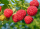Carrie picked 2/5 of the raspberries from the garden, and Robin picked some too.  When they were finished, 1/3 of the raspberries still needed to be picked.  What fraction of the raspberries did Robin pick? Use pictures, numbers or words and write your fi
• Complicated sum minus productWhat must be subtracted from the sum of 3/8 and 5/16 to get difference equal to the product of 5/8 and 3/16?
• Honey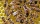Ila collected the honey from 3 of her beehives. From the first hive, she collected 2/3 gallons of honey. The last two hives yielded 1/4 gallon each. After using some of the honey she collected for baking, Lila found that she only had 3/4 gallon of honey l
• ChestnutsThree divisions of nature protectors participated in the collection of chestnut trees.1. the division harvested 1250 kg, the 2nd division by a fifth more than the 1st division and the 3rd division by a sixth more than the second division. How many tons of
• Lengths of the poolMiguel swam 6 lengths of the pool. Mat swam 3 times as far as Miguel. Lionel swam 1/3 as far as Miguel. How many lengths did Mat swim?
• Weigh in totalI put 3/5 kg of grapes into a box which is 1/4kg in weight. How many kilograms do the grapes and the box weigh in total?
• Math testBrayden was solving some math problems for the math team. He answered 2 math problems. Matthew answered 3, John answered 1 reasoning. Matthew 1/2 times as many. Brayden said that 2/6. Is he correct? Why or why not? Be sure to explain your answer.
• Submerging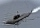Monika dove 9 meters below the ocean's surface. She then dove 13 meters deeper. Then she rose 19 and one-fourth meters. What was her position concerning the water's surface (the water surface = 0, minus values = above water level, plus = above water level
• Interior designer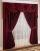To make draperies an interior designer needs 11 1/4 yards of material for the den and 8 1/2 yards for the living room. If material comes only in 20 yard bolts, how much will be left over after completing both sets of draperies?
• FrankFrank will be riding his bike to school this year. The distance from his house to the end of the street is ⅜ mile. The distance from the end of the street to the school is ⅚ mile. How far is Frank's house from school?
• Decimal to fractionWrite decimal number 8.638333333 as a fraction A/B in the basic form. Given decimal has infinite repeating figures.To three-eighths of one third, we add five quarters of one half and multiply the sum by four. How much will we get?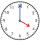Milada took the bus and the journey took 55 minutes. Jarmila was 1h 20 min by train. They arrived in Prague at the same time 10h45 min. At what time did each have to go out?